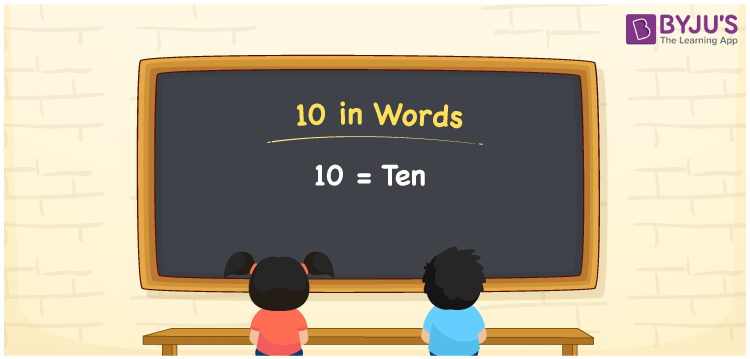# 10 in Words

10 in words can be written as Ten. The concepts such as count or counting and the importance of numbers can be understood effectively here. If you buy chocolate for Rs. 10 at the store, then you can say that “I bought chocolate for Ten Rupees at the store”. Learn more about how to write numbers in words with the English alphabet. Hence, the number 10 can be read as “Ten” in words.

 10 in words Ten Ten in Numerical Form 10

## 10 in English Words## How to Write 10 in Words?

In this article, you will get to know more about the place value chart for 10 in words. There are two digits in 10 and the place value for each digit is shown in the table below.

 Tens Ones 1 0

The expanded form of 10 is:

1 × Ten + 0 × One

= 1 × 10 + 0 × 1

= 10

= Ten

Therefore, 10 in words is written as Ten.

10 is a natural number that precedes 11 and succeeds 9.

10 in words – Ten

Is 10 an odd number? – No

Is 10 an even number? – Yes

Is 10 a perfect square number? – No

Is 10 a perfect cube number? – No

Is 10 a prime number? – No

Is 10 a composite number? – Yes

## Frequently Asked Questions on 10 in Words

Q1

### Write 10 in words.

10 can be written as “Ten” in words.
Q2

### What is 15 minus 5?

15 minus 5 is 10. Therefore, 10 in words is Ten.
Q3

### Is 10 a perfect square number?

No, 10 is not a perfect square number as it cannot be expressed as the product of two same integers.
Test your Knowledge on 10 in Words